# Induction motor:Condition for Maximum Starting Torque

## Condition for Maximum Starting Torque

It can be proved that starting torque is maximum when rotor resistance equals rotor reactance.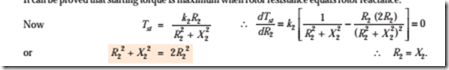#### Effect of Change in Supply Voltage on Starting Torque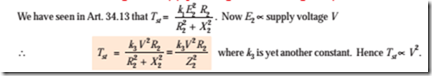Clearly, the torque is very sensitive to any changes in the supply voltage. A change of 5 per cent in supply voltage, for example, will produce a change of approximately 10% in the rotor torque. This fact is of importance in star-delta and auto transformer starters (Art. 33-11).

Example 34.6. A 3-6 induction motor having a star-connected rotor has an induced e.m.f. of 80 volts between slip-rings at standstill on open-circuit. The rotor has a resistance and reactance per phase of 1 W and 4 W respectively. Calculate current/phase and power factor when (a) slip-rings are short-circuited (b) slip-rings are connected to a star-connected rheostat of 3 W per phase.

##### (Electrical Technology, Bombay Univ. 1987, and similar example: Rajiv Gandhi Techn. Univ. Bhopal, Dec. 2000)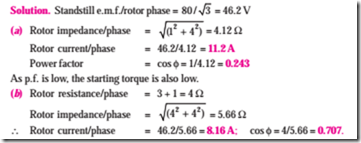Hence, the starting torque is increased due to the improvement in the power factor. It will also be noted that improvement in p.f. is much more than the decrease in current due to increased impedance.

Example 34.7. A 3-phase, 400-V, star-connected induction motor has a star-connected rotor with a stator to rotor turn ratio of 6.5. The rotor resistance and standstill reactance per phase are 0.05 W and 0.25 W respectively. What should be the value of external resistance per phase to be inserted in the rotor circuit to obtain maximum torque at starting and what will be rotor starting current with this resistance?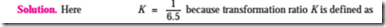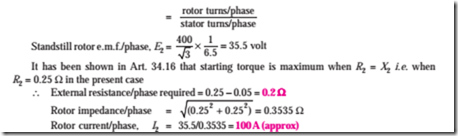Example 34.8. A 1100-V, 50-Hz delta-connected induction motor has a star-connected slip-ring rotor with a phase transformation ratio of 3.8. The rotor resistance and standstill leakage reactance are 0.012 ohm and 0.25 ohm per phase respectively. Neglecting stator impedance and magnetising current determine.

(iii) the rotor current at 4% slip with slip-rings shorted

(iv) the rotor power factor at 4% slip with slip-rings shorted

(v) the external rotor resistance per phase required to obtain a starting current of 100 A in the stator supply lines. (Elect. Machines AMIE Sec. B 1992)

Solution. It should be noted that in a D/Y connection, primary phase voltage is the same as the line voltage. The rotor phase voltage can be found by using the phase transformation ratio of 3.8 i.e. K = 1/3.8.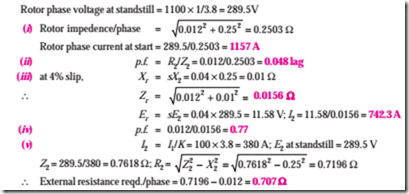Example 34.9. A 150-kw, 3000-V, 50-Hz, 6-pole star-connected induction motor has a star- connected slip-ring rotor with a transformation ratio of 3.6 (stator/rotor). The rotor resistance is 0.1W/phase and its per phase leakage reactance is 3.61 mH. The stator impedance may be neglected. Find the starting current and starting torque on rated voltage with short-circuited slip rings.

##### (Elect. Machines, A.M.I.E. Sec. B, 1989)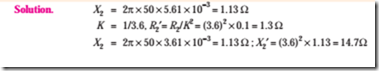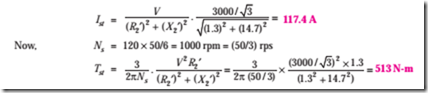Tutorial Problem No. 34.1

1. In the case of an 8-pole induction motor, the supply frequency was 50-Hz and the shaft speed was 735 r.p.m. What were the magnitudes of the following: (Nagpur Univ., Summer 2000)

(i) synchronous speed

(ii) speed of slip

(iii) per unit slip

(iv) percentage slip

[750 r.p.m. ; 15 r.p.m.; 0.02 ; 2%]

2. A 6-pole, 50-Hz squirrel-cage induction motor runs on load at a shaft speed of 970 r.p.m.

Calculate:-

(i) the percentage slip

(ii) the frequency of induced current in the rotor.

[3% ; 1.5 Hz]

3. An 8-pole alternator runs at 750. r.p.m. and supplies power to a 6-pole induction motor which has at full-load a slip of 3%. Find the full-load speed of the induction motor and the frequency of its rotor e.m.f.

[970 r.p.m. ; 1.5 Hz]

4. A 3-phase, 50-Hz induction motor with its rotor star-connected gives 500 V (r.m.s.) at standstill between the slip-rings on open-circuit. Calculate the current and power factor at standstill when the rotor winding is joined to a star-connected external circuit, each phase of which has a resistance of 10 W and an inductance of 0.04 H. The resistance per phase of the rotor winding is 0.2 W and its inductance is 0.04 H.

Also, calculate the current and power factor when the slip-rings are short-circuited and the motor is running with a slip of 5 per cent. Assume the flux to remain constant.

[10.67 A; 0.376; 21.95 A; 0.303]

5. Obtain an expression for the condition of maximum torque of an induction motor. Sketch the torque- slip curves for several values of rotor circuit resistance and indicate the condition for maximum torque to be obtained at starting.

If the motor has a rotor resistance of 0.02 W and a standstill reactance of 0.1 W, what must be the value of the total resistance of a starter for the rotor circuit for maximum torque to be exerted at starting ?

[0.08 W] (City and Guilds, London)

6. The rotor of a 6-pole, 50-Hz induction motor is rotated by some means at 1000 r.p.m. Compute

(i) rotor voltage (ii) rotor frequency (iii) rotor slip and (iv) torque developed. Can the rotor rotate at this speed by itself ?

[(i) 0 (ii) 0 (iii) 0 (iv) 0; No] (Elect. Engg. Grad I.E.T.E. June 1985)

7. The rotor resistances per phase of a 4-pole, 50-Hz, 3-phase induction motor are 0.024 ohm and 0.12 ohm respectively. Find the speed at maximum torque. Also find the value of the additional rotor resistance per phase required to develop 80% of maximum torque at starting.

[1200 r.p.m. 0.036 W] (Elect. Machines, A.M.I.E. Sec. B, 1990)

8. The resistance and reactance per phase of the rotor of a 3-phase induction motor are 0.6 ohm and 5 ohms respectively. The induction motor has a star-connected rotor and when the stator is connected to a supply of normal voltage, the induced e.m.f. between the slip rings at standstill is 80 V. Calculate the current in each phase and the power factor at starting when (i) the slip-rings are shorted, (ii) slip-rings are connected to a star-connected resistance of 4 ohm per phase.

[(i) 9.17 amp, 0.1194 lag (ii) 6.8 amp, 0.6765 lag][Rajiv Gandhi Technical University, Bhopal, 2000]

#### Incoming search terms: